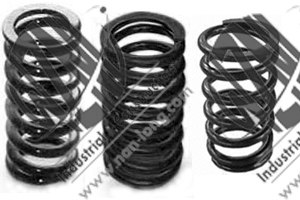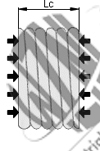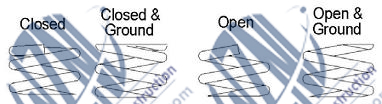# Compression Spring(Compressed Spring)|ChinaSpring >>

# Compression Spring#Physical parameters :

• Mean Diameter(D):is used in the stress and deflection calculations, and is equal to half the sum of the outside and inside diameters.
• Wire Diameter(d):.It is the diameter of the wire used to manufacture the spring and is a factor used to calculate spring index.
• Internal Diameter(ID): is specified when the spring will function over a rod or shaft . Internal diameter of a spring can be calculated by subtracting the doubled wire diameter from the external diameter of a spring.
• External Diameter(OD): is specified when the spring will function in a cavity External diameter of a spring can be calculated by adding the doubled wire diameter to the internal diameter of a spring.
• Pitch(P): the distance between wire centers in adjacent active coils. Current recommended practice is to specify the number of active coils rather than pitch.
• Block Length(LC): Maximal length of a spring after total blocking. This parameter is shown in the picture on right.
• free length(L0): the overall length of an unloaded (free) spring.
• Nr of coils: This is a total number of coils in a spring - in the picture above it is equal to 11. To calculate number of active coils substract two terminating coils from total number of coils.
Materials :
• Music Wire: Steel that conforms to the EN 10270-1 class SH standard.
• Inox steel: Inox 18/8 mark Z10 CN 18.09.
• Zn coated wire: Zinc coated wire for springs
• Cr-Si Steel: Cr-Si Steel. Springs created from this material have better endurance and spring rate.
Characteristics :
• Ends: There are several kinds of the ends:Squared(Closed) one,Square(Closed) & Ground one, Plain(Open) one,Plain(Open)& Ground One.Please see them as follow• Spring rate: Tolerance for spring rate is in resonable range or customer's enquiry.